# How do nuclei rotate 1 3 symmetry axis

• Slides: 16
Download presentation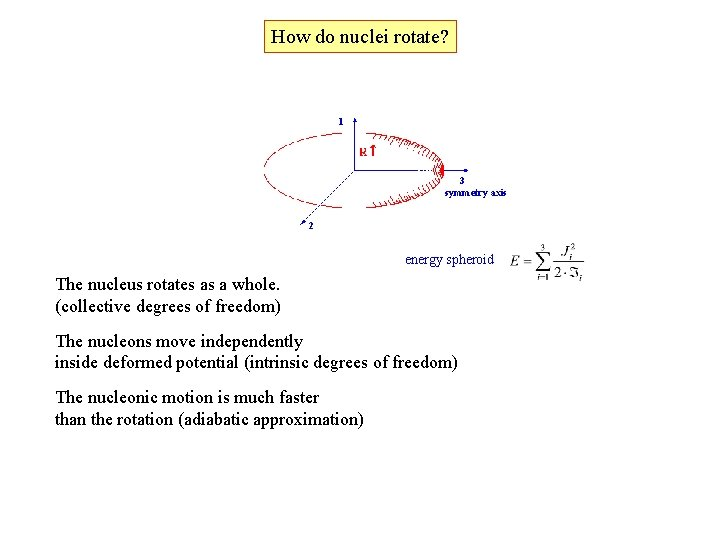How do nuclei rotate? 1 3 symmetry axis 2 energy spheroid The nucleus rotates as a whole. (collective degrees of freedom) The nucleons move independently inside deformed potential (intrinsic degrees of freedom) The nucleonic motion is much faster than the rotation (adiabatic approximation)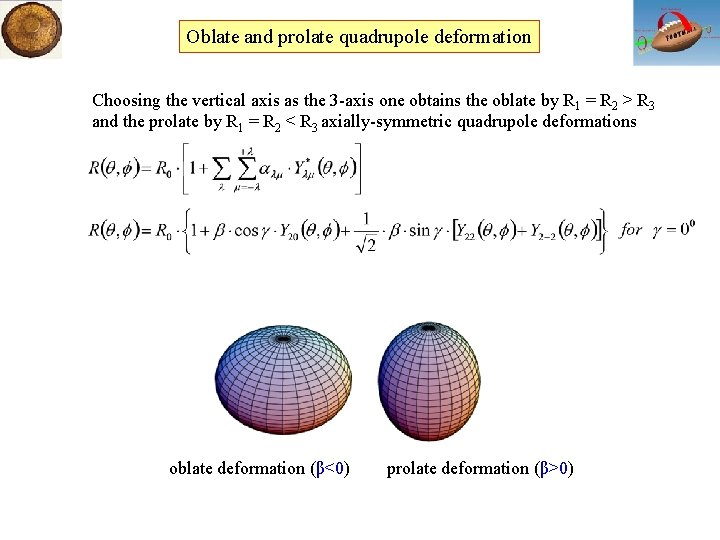Oblate and prolate quadrupole deformation Choosing the vertical axis as the 3 -axis one obtains the oblate by R 1 = R 2 > R 3 and the prolate by R 1 = R 2 < R 3 axially-symmetric quadrupole deformations oblate deformation (β<0) prolate deformation (β>0)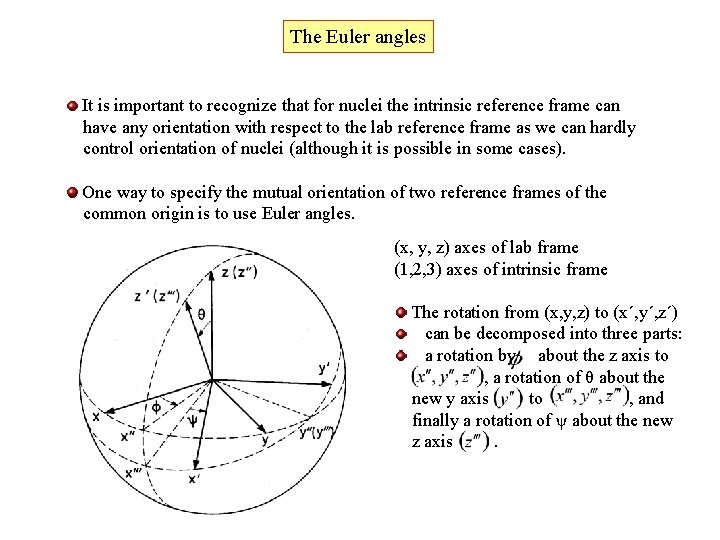The Euler angles It is important to recognize that for nuclei the intrinsic reference frame can have any orientation with respect to the lab reference frame as we can hardly control orientation of nuclei (although it is possible in some cases). One way to specify the mutual orientation of two reference frames of the common origin is to use Euler angles. (x, y, z) axes of lab frame (1, 2, 3) axes of intrinsic frame The rotation from (x, y, z) to (x´, y´, z´) can be decomposed into three parts: a rotation by about the z axis to , a rotation of θ about the new y axis to , and finally a rotation of ψ about the new z axis.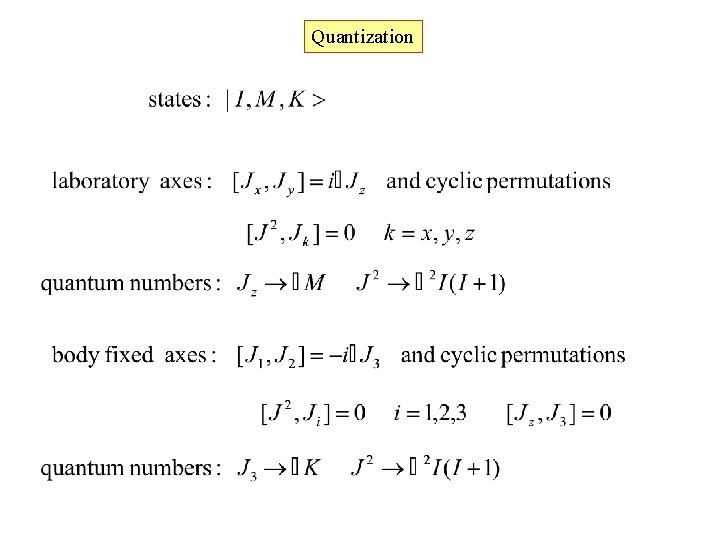Quantization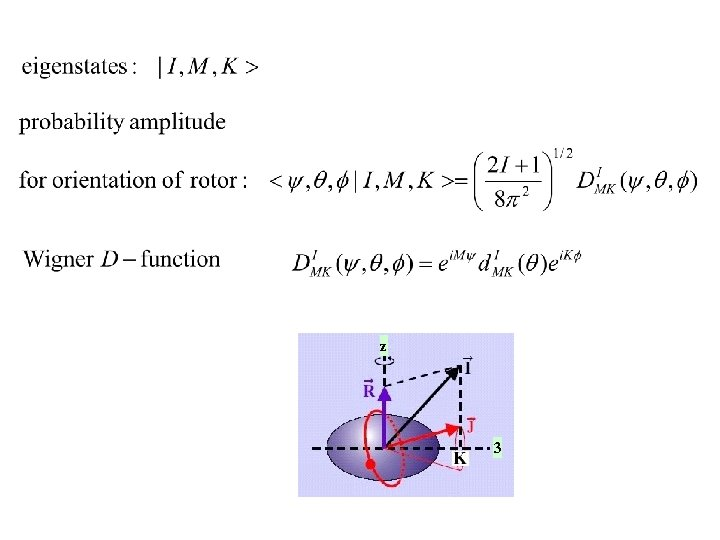z 3Rotational motion of a deformed nucleus z For a deformed nucleus with axial symmetry one obtains the same rotational frequency for the 1 - and 2 -axis. The Hamiltonian is given by 3 The nucleus does not have an orientation degree of freedom with respect to the symmetry axis States with projections K and –K are degenerated This fact is expressed in the nuclear wave function as a symmetric product J For K=0, only even-J are allowed, so that the wave function is given by a single term 3 If the total angular momentum results only from the rotation (J = R), one obtains for the rotational energy of an axially symmetric nucleus byproton number Broad per spective on structural evolution: neutron number Note the characteristic, repeated patterns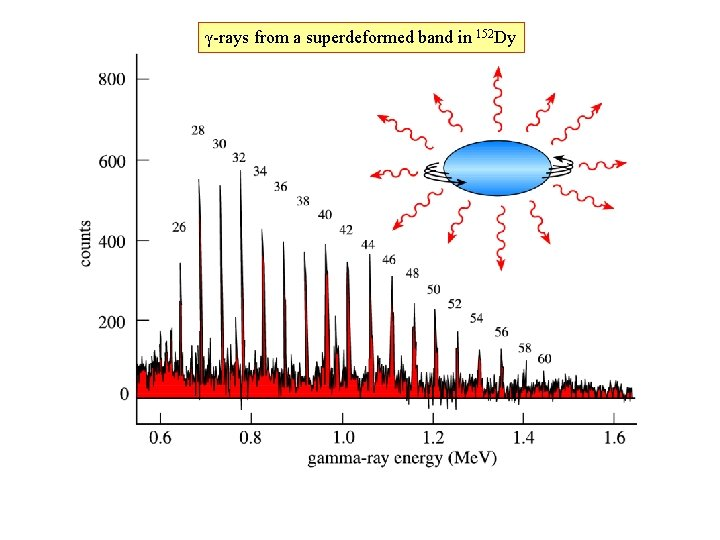γ-rays from a superdeformed band in 152 Dy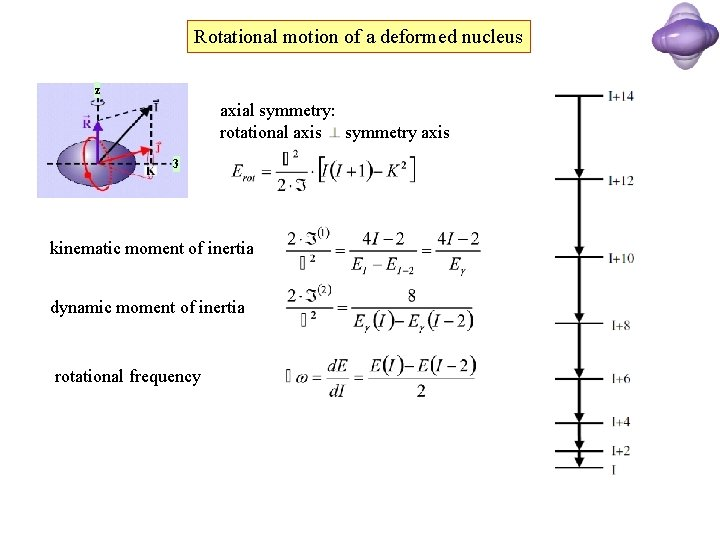Rotational motion of a deformed nucleus z axial symmetry: rotational axis symmetry axis 3 kinematic moment of inertia dynamic moment of inertia rotational frequencyMoment of inertia z R( ) Rigid body moment of inertia: Irrotational flow moment of inertia:Moment of inertia rigid irrotationalReduced transition probability expectation value wave function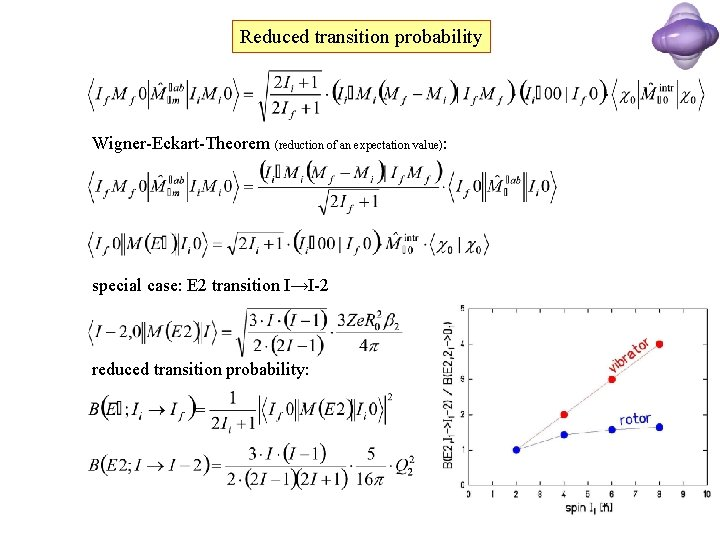Reduced transition probability Wigner-Eckart-Theorem (reduction of an expectation value): special case: E 2 transition I→I-2 reduced transition probability: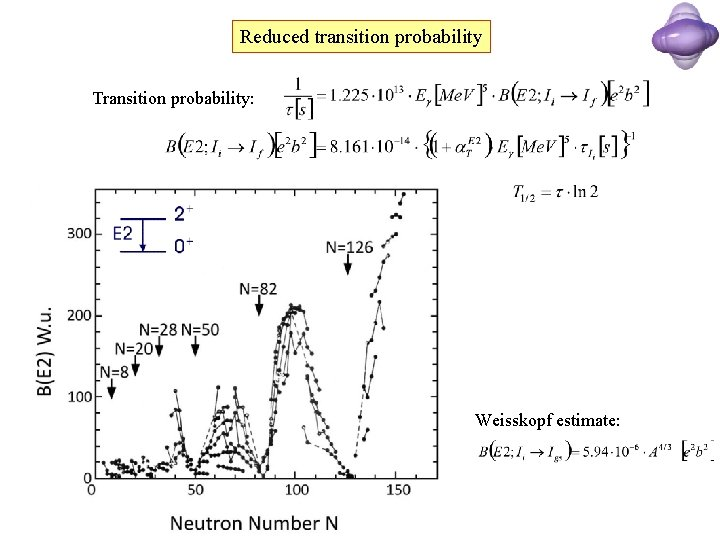Reduced transition probability Transition probability: half-life: Weisskopf estimate: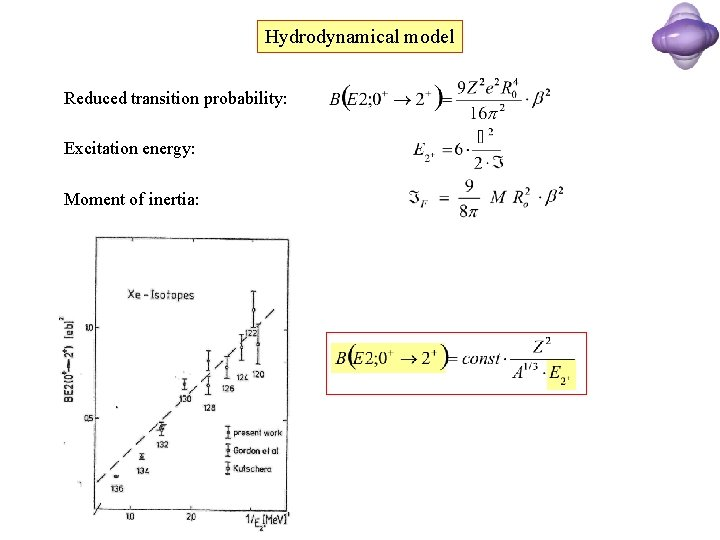Hydrodynamical model Reduced transition probability: Excitation energy: Moment of inertia: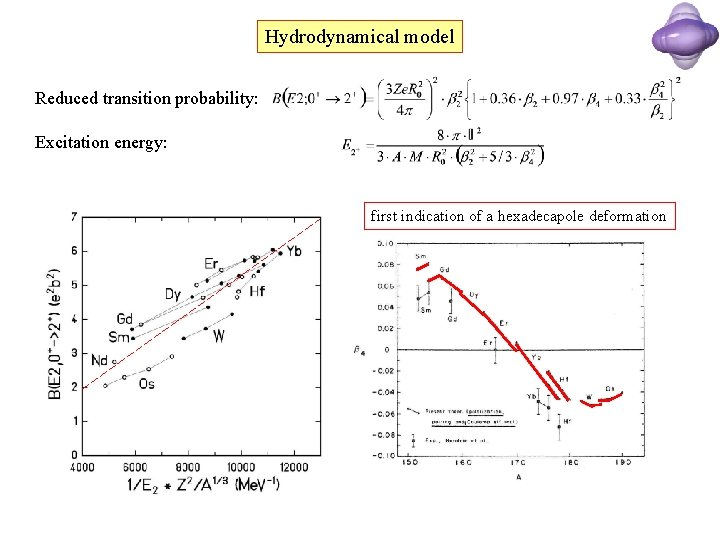Hydrodynamical model Reduced transition probability: Excitation energy: first indication of a hexadecapole deformation